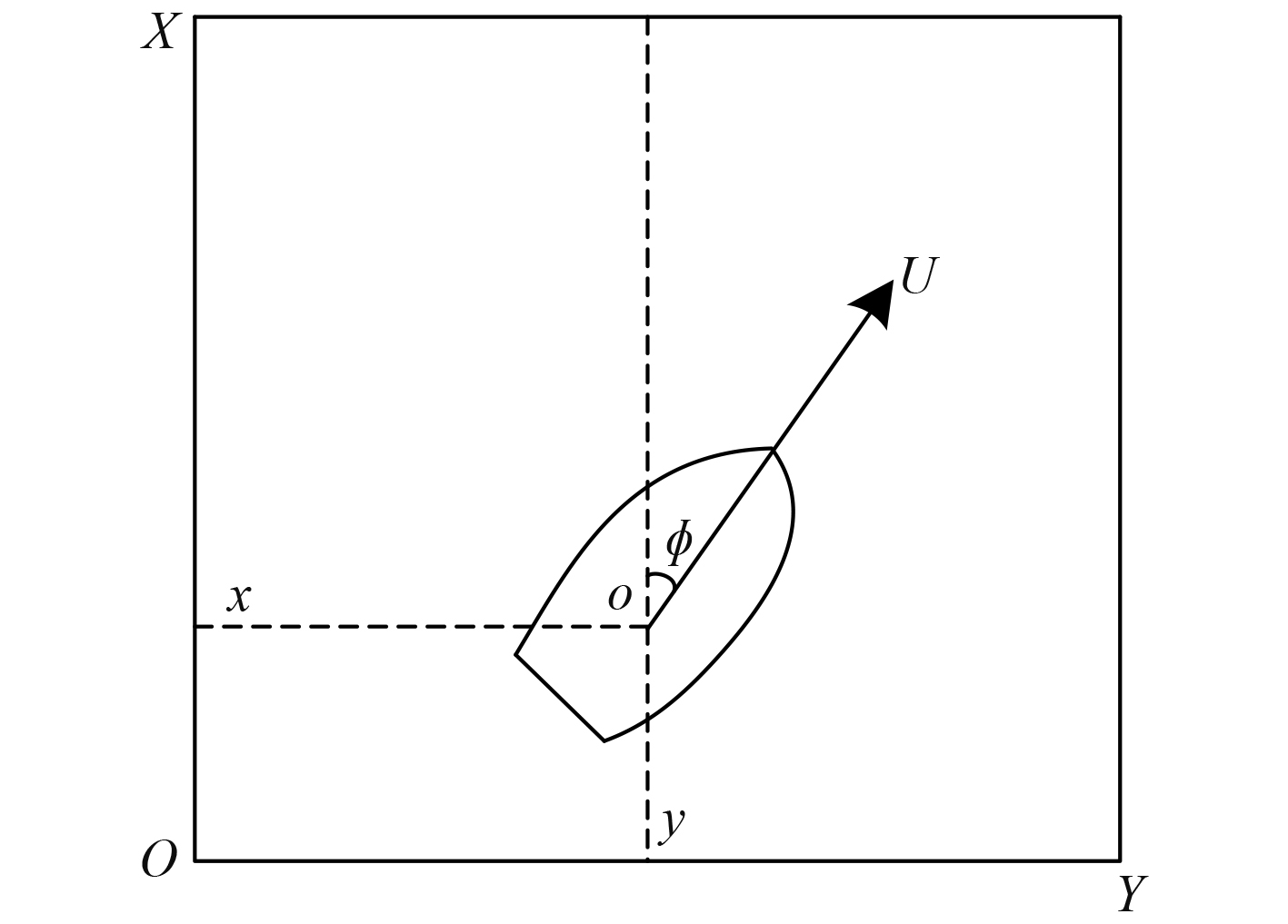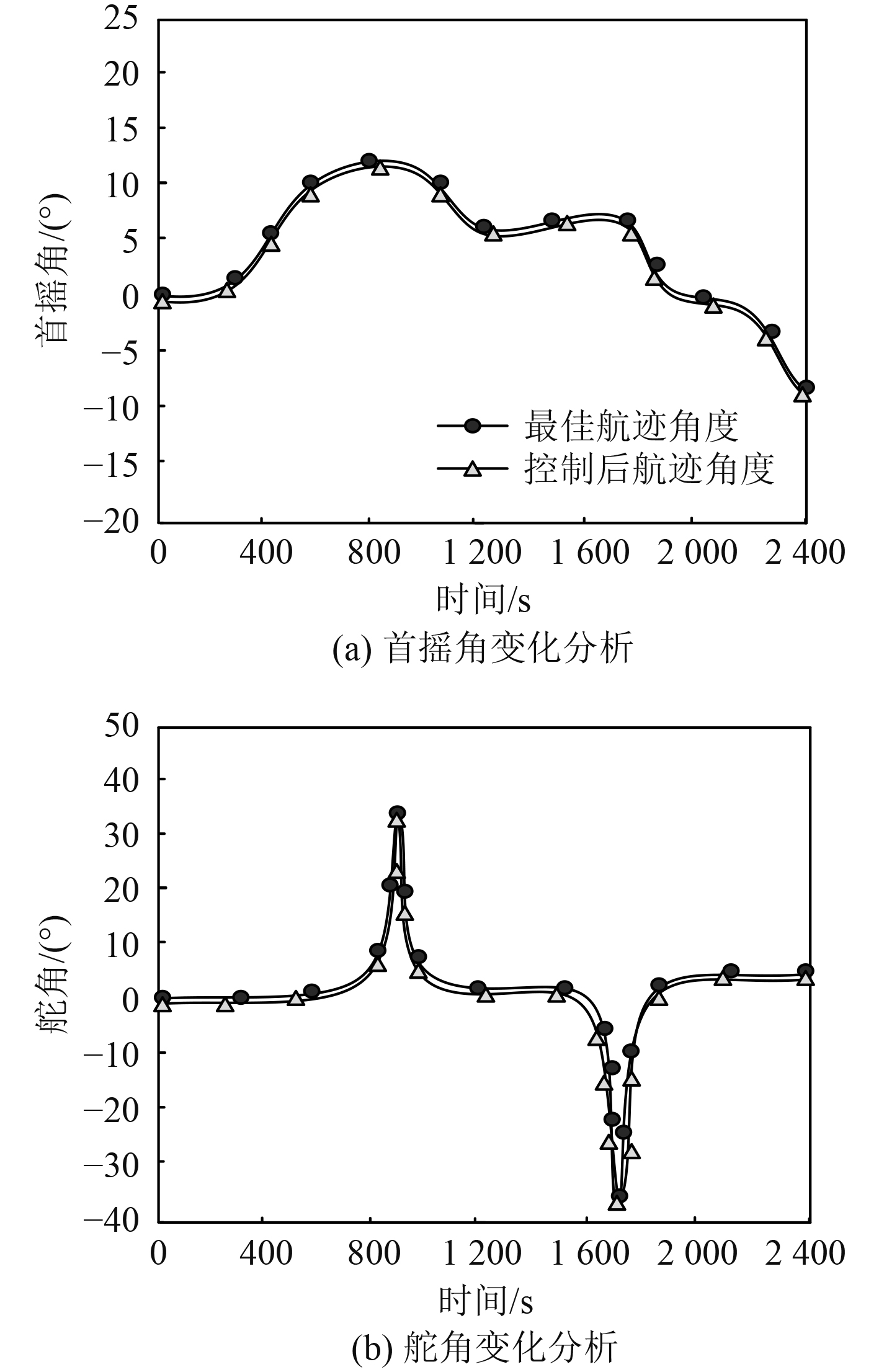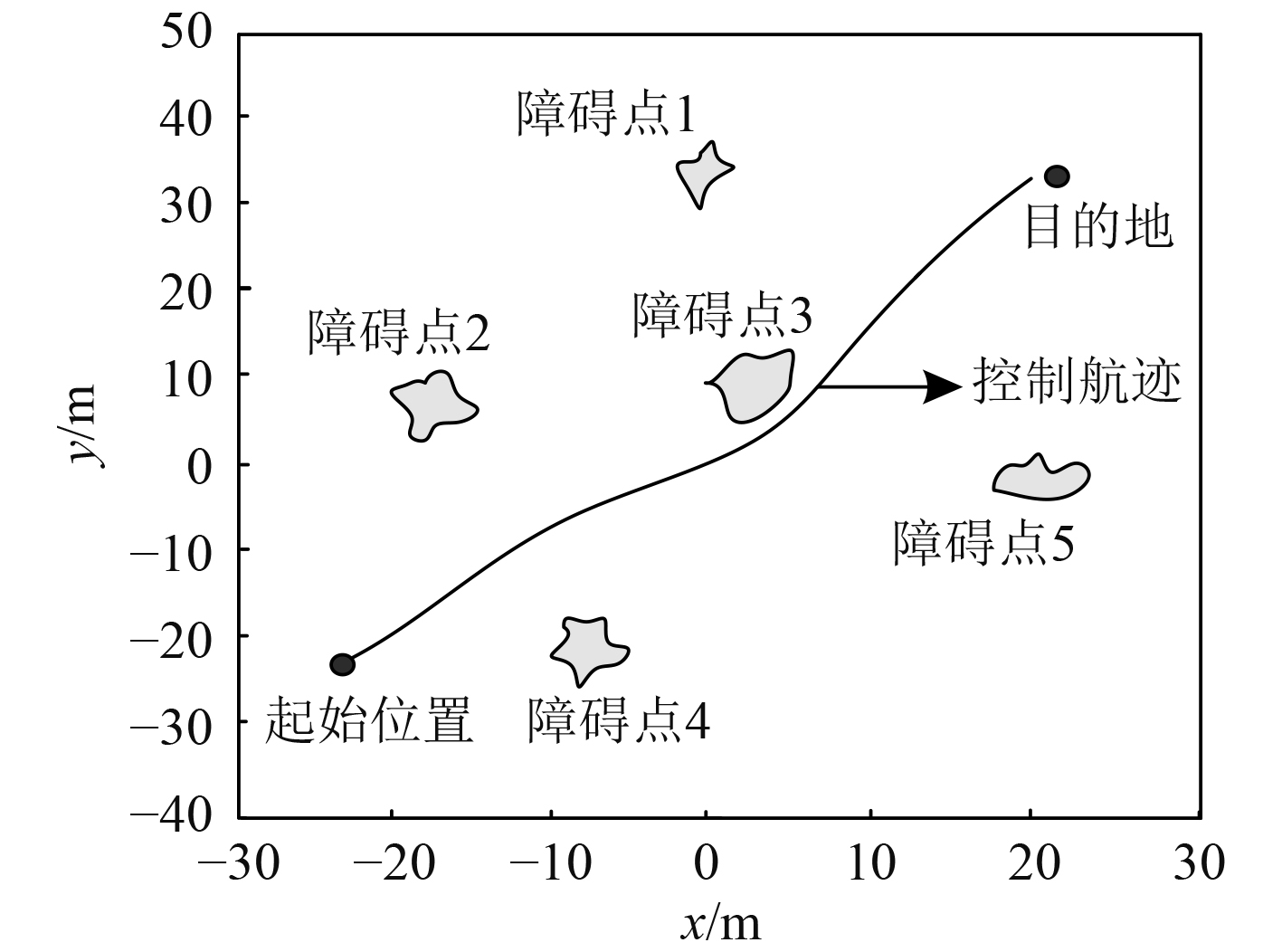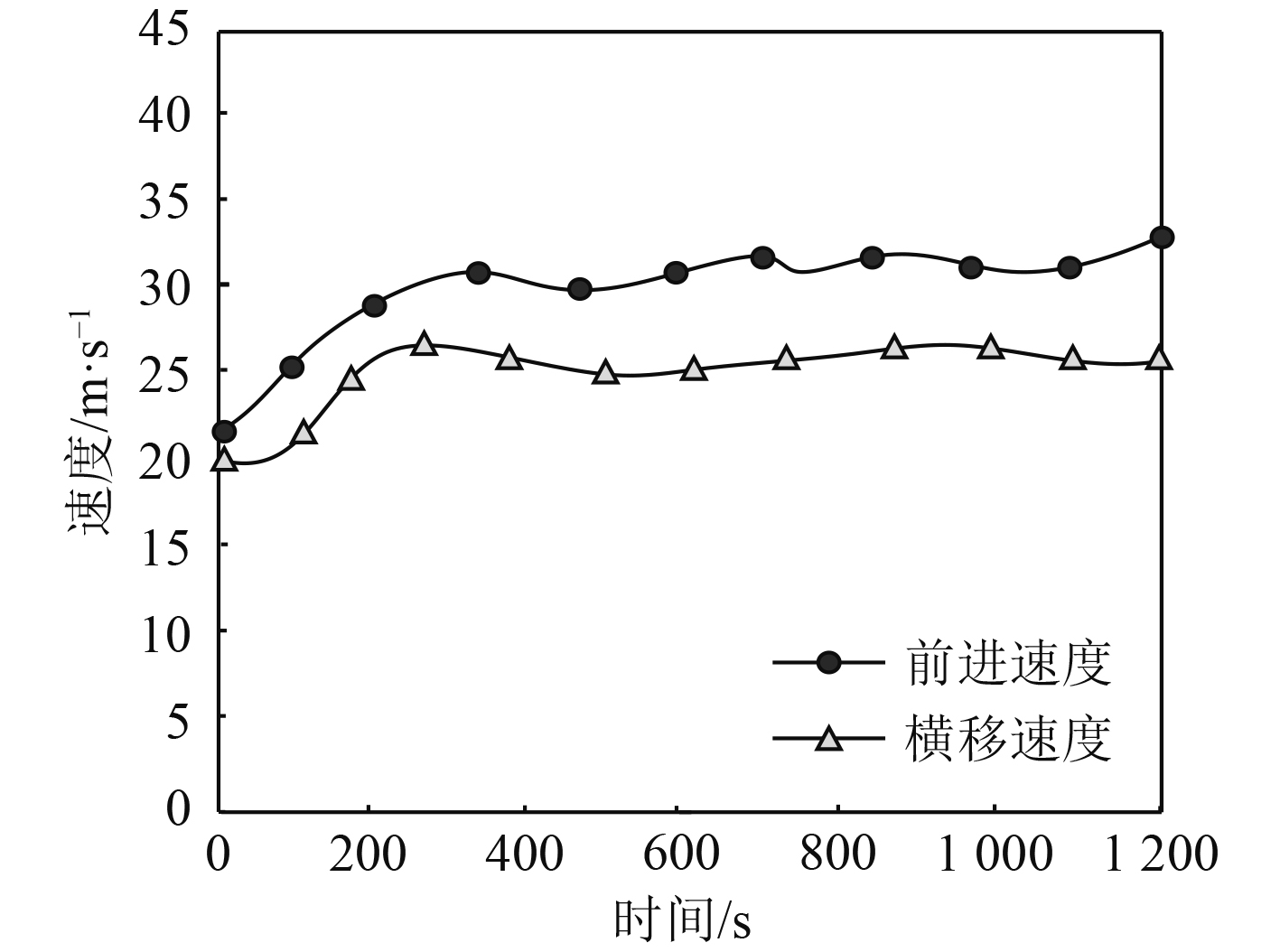﻿ 基于关联规则的船舶航迹分布式控制方法
 舰船科学技术2022, Vol. 44Issue (14): 65-68    DOI: 10.3404/j.issn.1672-7649.2022.14.015PDF

Distributed control method of ship track based on association rules
CHEN Ting-ting, RUI Le-jun
Jiangsu Shipping College, Nautical Department, Nantong 226010, China
Abstract: In order to provide reliable security for ship navigation, a distributed ship track control method based on association rules is designed. The multi factor association rules of ship navigation are introduced to search the ship track based on the shortest mileage and the shortest sailing time. The distributed control mathematical model of ship track is constructed. The optimal sailing track is input into the model to realize the fixed sailing track control. The distributed backstepping controller of ship track is designed to realize the distributed control of linear changing ship track. The experimental results show that this method can effectively control the ship's yaw angle and rudder angle, and can realize the ship's angle control according to the best track, and the ship's forward speed and traverse speed are improved after control.
Key words: association rules     ship's track     distributed control     bow wave angle     shortest mileage     push-back controller
0 引　言

1 船舶航迹分布式控制设计 1.1 基于关联规则A*改进算法的船舶最佳航迹搜索 1.1.1 基于关联规则A*改进算法

A*算法是一种较早应用在数据挖掘领域的关联规则算法，利用基于关联规则的A*改进算法，搜索最佳船舶航迹，为航迹分布式控制提供可靠基础。最佳航迹搜索时考虑多约束的A*算法代价函数模型，即

 $f\left( n \right) = \sum\limits_{i = 1}^N {\sum\limits_{j = 1}^N {{w_{ij}}{x_{ij}}} } 。$ (1)

1.1.2 算法搜索船舶最佳航迹流程

1.2 船舶航迹分布式控制数学模型图 1 航迹控制坐标 Fig. 1 Track control coordinates

1.3 船舶航迹分布式反推控制器设计 1.3.1 输入-状态反馈线性化

 $\dot x = f\left( x \right) + g\left( x \right)u 。$ (2)

 $\dot z = Az + B\vartheta \left( x \right)v。$ (3)

1.3.2 反推船舶航迹分布式控制器

 ${\dot V_1} = {z_1}{z_2} = - {\vartheta _1}z_1^2 + {z_1}{\tilde z_2} 。$ (4)

${\dot V_1}$ 为微分后的Lyapunov函数 ${V_1}$ ，可将式(3)中的前2个状态方程调整为：

 $\left\{ \begin{gathered} {{\dot z}_1} = - {\vartheta _1}{z_1} + {{\tilde z}_2}，\\ \dot {\tilde z} = - \vartheta _1^2{z_1} + {\vartheta _1}{{\tilde z}_2} + {z_3}。\\ \end{gathered} \right.$ (5)

 ${z_{3d}} = \left( {\vartheta _1^2 - 1} \right){z_1} - \left( {{\vartheta _1} - {\vartheta _2}} \right){\tilde z_2} 。$ (6)

 ${V_2} = {V_1} + \frac{1}{2}\tilde z_2^2 。$ (7)

 ${z_{3d}} = - {h_1}{z_1} - {h_2}{z_2}。$ (8)

 $\left\{ \begin{gathered} {{\dot z}_1} = {z_2} ，\\ {{\dot z}_2} = {z_3} 。\\ \end{gathered} \right.$ (9)

${\tilde z_3} = {z_3} - {z_{3d}}$ ，此时可将航迹分布式控制模型式(2)变换为以下形式：

 $\left\{ \begin{gathered} {{\dot {\tilde z}}_1} = {z_2}，\\ {{\dot {\tilde z}}_2} = {{\tilde z}_3} + {z_{3d}}，\\ {{\dot {\tilde z}}_3} = {h_1}{z_2} + {h_2}{z_{3d}} + {h_2}{{\tilde z}_3} + v\left( t \right)。\\ \end{gathered} \right.$ (10)

 $v\left( t \right) = - {\vartheta _3}{\tilde z_3} - {h_2}{\tilde z_3} - \left( {{\vartheta _1} - {h_1}{h_2}} \right){z_1} - \left( {1 + {h_1} - h_2^2} \right){z_2} 。$ (11)

2 实验结果分析表 1 航迹规划情况分析 Tab.1 Analysis of track planning图 2 航角变化控制能力分析 Fig. 2 Analysis of control ability of angular change图 3 航迹控制情况分析 Fig. 3 Analysis of track control图 4 行驶速度变化情况 Fig. 4 Variation of driving speed
3 结　语

  刘文, 汪文博. 基于秩最小化矩阵去噪的船舶轨迹重构方法[J]. 交通运输系统工程与信息, 2022, 22(1): 106-114. DOI:10.16097/j.cnki.1009-6744.2022.01.012  高大为, 朱永生, 张金奋, 等. 基于AIS数据的船舶航迹多维预测方法[J]. 中国航海, 2021, 44(3): 56-63. DOI:10.3969/j.issn.1000-4653.2021.03.010  崔彤彤, 王桂玲, 高晶. 基于1DCNN-LSTM的船舶轨迹分类方法[J]. 计算机科学, 2020, 47(9): 175-184. DOI:10.11896/jsjkx.191000162  刘彦呈, 许晨, 赵友涛, 等. 基于超螺旋滑模的欠驱动船舶航迹跟踪控制[J]. 信息与控制, 2020, 49(5): 578-584+597. DOI:10.13976/j.cnki.xk.2020.9651  赵顺利, 李伟, 张文拴. 基于径向基函数神经网络的船舶航迹自抗扰控制[J]. 上海海事大学学报, 2020, 41(4): 20-24.  张黎翔, 朱怡安, 陆伟, 等. 基于AIS数据的船舶轨迹修复方法研究[J]. 西北工业大学学报, 2021, 39(1): 119-125. DOI:10.3969/j.issn.1000-2758.2021.01.015  祝亢, 黄珍, 王绪明. 基于深度强化学习的智能船舶航迹跟踪控制[J]. 中国舰船研究, 2021, 16(1): 105-113.  祁林, 渠俊锋, 司文杰, 等. 基于RBF神经网络的水面船舶轨迹跟踪控制[J]. 船舶工程, 2021, 43(1): 95-101+118. DOI:10.13788/j.cnki.cbgc.2021.01.17  刘娇, 史国友, 杨学钱, 等. 基于DE-SVM的船舶航迹预测模型[J]. 上海海事大学学报, 2020, 41(1): 34-39+115.